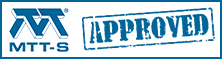# A Note on Noise

New for January 2019.  This page was written by Terry, and has been reviewed and approved by IEEE MTT-S.  Thanks!

Noise is a confusing term. Actually, it’s not hard to see why. Understanding it means quantifying what we mean by random and chaotic behavior.  Fundamentally, noise corrupts, masks, hides the information it coexists with. How are we to deal with this issue? Well, pretty much like we deal with the process of designing a circuit or chip. The process begins with knowing and understanding the behavior of basic electronic components – like resistors, capacitors, transmission lines etc.

Next we need a method of combining these individual behaviors to predict the aggregate behavior of the entire circuit or device that we are designing. This theory exists and is well advanced and resident in the CAD tools we use to simplify the circuit analysis. Consequently, we can concentrate on creating the performance we desire and not be bogged down by the details of the calculation process. Further, we can use the fundamental results of these tools, like currents and voltages, to translate them into sets of standard input-output performance parameters – gain, power, efficiency etc. – and into Network Parameters that allow the results of one circuit analysis to be combined with the results of another independent circuit analysis. It happens that it only requires four complex variables (parameters) to describe the aggregate behavior of all of the interconnected components of a specific linear network at any given frequency using Network Parameters like S-parameters, Y-parameters, ABCD-parameters, etc.

It turns out that we can similarly predict the aggregate behavior of a noisy circuit at a given frequency using only 2 real variables plus 1 complex variable (4 values). In 1956, in the first step in this direction, Rothe and Dahlke showed that we can replace any linear noisy two-port circuit by an identical noiseless circuit preceded by a series-parallel combination of a noisy voltage source and a noisy current source which in general may have some degree of correlation between them, despite the two sources representing random processes. The circuit performance of the resulting combination is identical to the original circuit behavior. Note also that the sources labeled en and In are statistical voltage and current sources whose RMS voltages and currents are proportional to noise power. The two noise sources are at the input to the lossless network and are called input referred noise sources, since all the interior noise sources have been combined and translated to the input.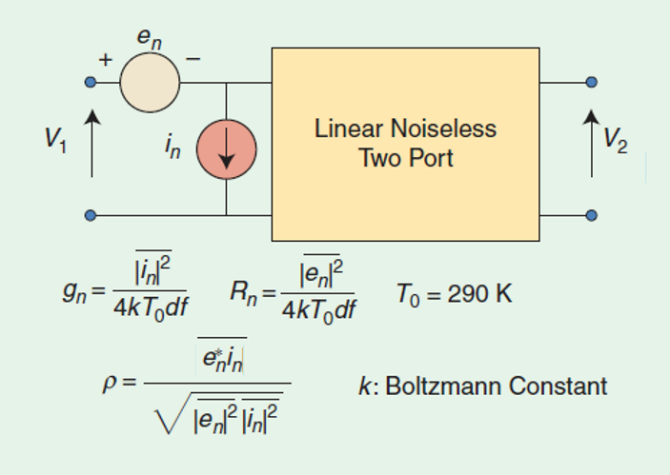As a further note, when I’m talking about noise I’m focusing on random fluctuations of electrical charges within a system or network and not those sources of noise that are not native and are instead signals coupled into a system – like the Cosmic Microwave Background radiation from the Big Bang and your neighbor’s microwave oven. I also exclude fluctuations created by the quantization of a signal.

Noise

Following the same path pioneered by linear circuit analysis, we begin by identifying sources of noise and ways to quantify the behavior of these random processes. The noise contribution from individual circuit components is usually defined in statistical terms. If we look at a noisy signal, we see a random variation in voltage or current amplitude over a range of frequencies. The average of this signal is zero, but the RMS value over time is not zero – just as average of a sine wave is zero, the average of the square of the sine wave is not zero. Consequently, we usually concern ourselves with noise power or, more precisely, noise spectral power density (power per unit bandwidth.)

Like the network parameters of a linear circuit analysis (power, gain, etc.), we define noise network parameters such as noise figure, noise factor and noise temperature. These are terms that quantify the amount of noise created within a network.

The ratio of the available signal power to the unwanted available noise power (SNR) is an obvious performance metric quantifying the degree to which the noise is corrupting the coexisting desired signal. The noise factor (F) of a circuit is another metric and is probably the most frequently used parameter to describe the behavior of a circuit. It is defined as the signal-to-noise ratio at the input to the circuit divided by the signal-to-noise ratio at the output. The noise factor is always greater than unity, as long as the measurement bandwidth is the same for the input and output data. A network without any noise sources will have a noise factor of one. The noise factor of a component measures the degree to which that component creates additional noise to make it harder to see the desired signal. One of the major goals of this discussion is to show the significance of this performance metric and how to compute it for a given circuit.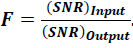Noise Figure (NF) is the Noise Factor expressed in dB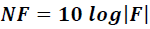For passive devices, the computation of NF is trivial because it is identical to the loss of the device in dB. The definitions for noise figure and noise factor are valid for any electrical network, including frequency conversion networks that contain mixers and IF amplifiers (up-converters or downconverters).

Johnson Noise

Temperature is a way of quantifying an ensemble behavior like the behavior of particles in a gas. Noise voltage is another way of specifying or quantifying randomness such as the random movements of electrical charges within an electronic component. Boltzman’s constant (k= 1.38065E-23 joules per kelvin) is a statistical bridge between macroscopic and microscopic physics that helps us get started in understanding and quantifying noise.

In one of the most significant papers of 1928, Nyquist, using the equipartition law of thermodynamics, showed that a thermodynamic system consisting of a resistor, R, at thermodynamic equilibrium and at an absolute temperature of T (Kelvin) could be characterized as an RMS Gaussian noise voltage in which power is related to the voltage, temperature and bandwidth by: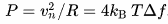It is a startling fact that this RMS noise voltage depends neither on the material nor the configuration of the electrical circuit. Instead, the expression governing it is relatively simple and depends only on temperature, bandwidth and Boltzmann’s constant. It also means that we can simulate the noise behavior of a noisy circuit using standard circuit analysis after replacing each noisy resistor with a noiseless resistor of the same value and its associated series connected RMS voltage source.

Finally, notice that Johnson noise power is independent of the value of the associated resistor and frequency!

After a little thought this seems a bit silly. A resistor lying on a table is generating power? The trick is that the resistor is in thermal equilibrium (I slipped that in a few sentences ago), and so it is receiving as much energy as it is dissipating. This type of noise was discovered and first measured by John B. Johnson at Bell Labs in 1926. He described his findings to Harry Nyquist, also at Bell Labs, who was able to explain the results. There is a Norton equivalent RMS current expression using current and conductance in place of voltage and resistance.

The IEEE standard for room temperature is 290 Kelvin (not 300K or 273.15K or 291.15K). Hence at the “standard temperature, To,” of 290 Kelvin, the thermal noise power (kTB) for a 1 Hz bandwidth is 4.005E-21 W or -173.98 dBm – assuming a flat (white) noise power spectrum versus frequency. (Thanks to Paolo for the correction, January 2020!)

Another way of looking at the Johnson noise power equation is to notice that for a given bandwidth and noise power, we can define a unique temperature directly proportional to the noise power spectral density (P/B) with P as noise power and B and the bandwidth. We can call this the effective noise temperature of a component with a noise factor of F, nomenclature in common use.

Te = 290(F-1)

Shot Noise

While thermal noise is the dominant source in most systems, it is not the only type of noisy fluctuation in our electronic circuits. Another different type of noise in electronic circuits is called Shot noise, where the current flow is due to the transport of discrete electrons. A Poisson fluctuation in their kinetic energy will modulate the arrival rate of charge carriers at the circuit node where current is measured. William Schottky defined this current based noise by the variance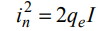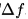Shot noise and Johnson noise are the most common source of noise in most electronic circuits. Both are “white noise sources,” independent of frequency, but they are by no means the only sources of noise.

Transistor Channel Noise

FET and other transistors have a thermal current noise source dependent on the device channel saturation properties and it is similar to Johnson noise using gm as the conductance and gamma as a constant that varies in value between 0.67 and 1.5.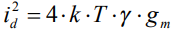Flicker Noise

Another type of noise is called flicker noise or 1/f noise or pink noise. Flicker noise exhibits an inverse frequency power density curve and is often characterized by the corner frequency fc between the region dominated by the low-frequency flicker noise and the higher-frequency "white noise" noise.

Flicker noise power of a device is described by an RMS current that is directly proportional to the dc (or average) current (i) flowing through the device as well as its associated corner frequency: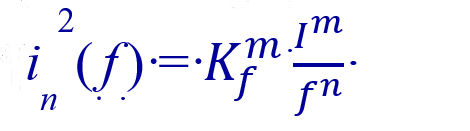where Kf  is a constant that depends on the type of material and 1 < m < 3, and 1 < n < 3.

Quantum Noise

There is something called the “quantum” noise limit which determines the lowest value of the noise temperature Tn of a high-gain "linear amplifier"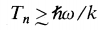defined by Planck’s constant, Boltzman’s constant and the operating frequency. This means that a "linear amplifier" must add noise, however small, to any signal it processes. At 10 GHz this amounts to about 500 mKelvins in terms of effective noise temperature – sounds pretty hard to measure.

We can graphically illustrate the behavior of a typically noisy component with thermal, shot, flicker and quantum sources of noise as shown below.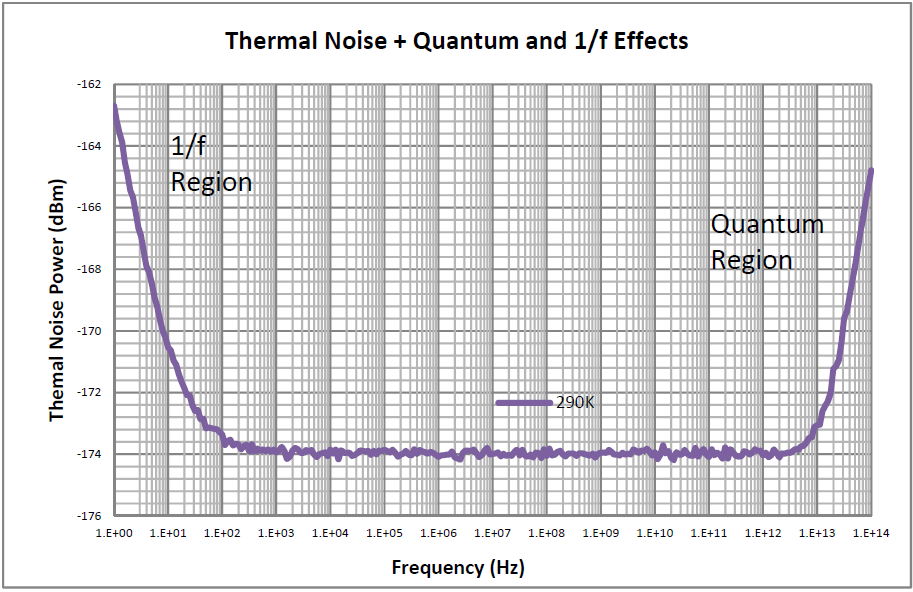Noise Parameters

Just as in circuit analysis, where the aggregate behavior of a collection of individual electronic components can be described at each frequency by a single set of Network Parameters (e.g. S-parameters, ABCD, Z, and Y parameters), the aggregate noise behavior of a collection of interconnected noisy components can be described by a set of data called Noise Parameters.

There are many equivalent sets of Optimum Noise Parameters and they all produce the same results. The most common set contains Fmin, Rn, and Optimum Source Match (complex), and another equivalent set contains Tmin, N, and Optimum Source Match (complex). These parameters allow you to predict the noise behavior of your entire circuit instead of having to compute all the individual noise powers and then translate them into the resulting aggregate noise performance. Source match refers to Gammaopt or Yopt or Zopt. From a design point of view this tells you that your design produces the optimum noise factor if it presented with a source impedance of Zopt. Further, it computes the noise factor of our circuit for any source impedance presented to its input.

Most of the time we are designing something that requires active devices (e.g. transistors) to achieve our design goals. Noise parameters help you to compare transistor device options and decide which transistor to use and then how to optimize for noise performance of the circuit in which the transistors are to be embedded. The noise factor is the metric that quantifies the noise performance of our network, so we need to determine how it is related to the noise parameters to be able to use them in our designs.

Noise Factor (Noise Figure)

We care about noise because it tends to corrupt or mask the weak signals that we wish to receive or process. Clearly, if the power level of the input signal we wish to use is comparable to the power level of the comingled noise, then we have a problem discerning the desired signal. Since every electrical component in a circuit design is either a lossy element or a lossless element that shapes the frequency response, the output SNR of the circuit is reduced and the noise factor based on the definition below is increasedwhere in the definition of noise factor, the input noise power density is always kTo, or ~-174 dBm/Hz and To equals 290 K and the signal-to-noise ratio is defined as the ratio of the power of a signal (meaningful information) to the power of background noise (unwanted signal). Sensitivity is another network parameter. It indicates the minimum input signal power required to produce a specified output signal having a specified signal-to-noise ratio, or some other specified criteria.

The block diagram below allows us to study the noise factor F.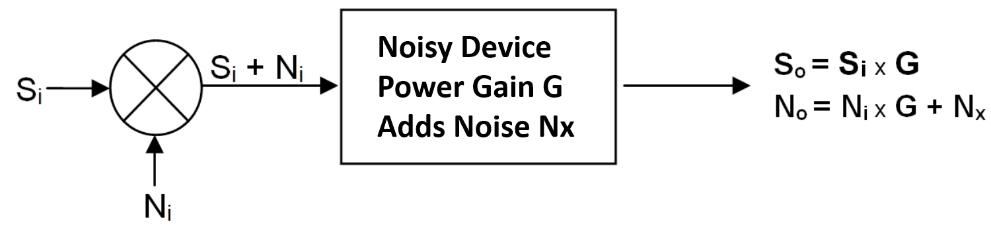Substituting these variables into the noise factor equation results in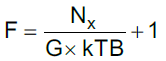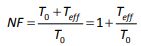where Ni = kToB by definition and Nx is the noise added by the device and Nx= kTeffB.

Here is some great news. You do not have to calculate or measure anything to know the noise figure of a passive linear network. It is just 1/G (insertion loss). Also note that the gain expression is “available power gain,” not the common Transducer Power Gain (S21). Available Gain is the ratio of the Power out of the network when it has a conjugate output load match to the power available from the source and Available Gain always related to a specific source impedance. Noise calculations use available power and, frequently, this noise power is referred to the input by dividing the output noise power by the available gain.

We can rewrite the noise factor equation to highlight the use of available output noise referred to the input (Nout,i = Nx/G ). The result is a noise factor defined as one plus the input referred noise power divided by the input noise power.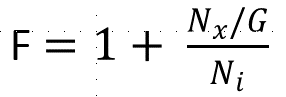Optimum Noise Factor

We all want to design the best circuits we can, and in 1969 in another significant paper on noise behavior, R L Lane derived an expression that relates the fundamental Noise Parameters Fmin, Rn, and YsOpt to the noise factor of the device at a given frequency and for a specific input admittance Ys. This gives us a wonderful tool to compute the source impedance we need to present to an active device to optimize the circuit noise factor.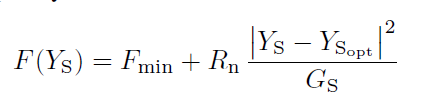Alternatively, the noise factor can be specified by noise temperatures, which in some cases is more convenient.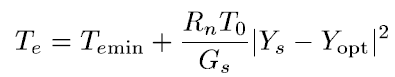where a constant value of for Te, or equivalently Fmin, results in a locus of Input Admittances (Ys) for a specific network that forms a circle in a Smith chart with the optimum input admittance, Yopt, at the center of the circle. If you combine noise circles and gain circles on a single Smith Chart, the overlap of these circles will give you a graphic visualization of the range of input Admittances that simultaneously satisfy specific gain and noise performance. This graphic display quickly shows the design trade-offs between noise and gain. Naturally, it is unlikely that the optimum noise performance source match will also be the optimum source match for gain – especially over a range of frequencies.

Evaluating the Noise Parameters

There are at least two ways to establish the Noise Parameters of a network or transistor:

One is to make four noise factor measurements at each frequency in which each measurement uses a different known input source admittance. Use that data to solve the Lane formula for the 4 unknown Noise Parameters at each frequency.

The second way is a brute force method, in which you replace all the noisy elements of a network with noiseless elements and their respective noise power sources, and then use circuit analysis to compute the signal and noise powers needed to determine the SNRs needed to compute the noise factor for a given Ys. If this is an algebraic expression, the derivative of the expression will lead the optimum Noise Parameters.

A third way is to use circuit analysis to refer the individual noise sources to the input, noting that any noisy linear two-port network can be simplified to a noiseless network with a single current and a single voltage noise source, although the two sources could be correlated to each other. The circuit analysis needed to refer the noise sources to the input can frequently use the Thevenin and Norton theories to find the equivalent input referred noise sources and their correlation.Once you have calculated the in and en sources based on the noisy circuit, gn, Rn, and ρ are known. From these values we can compute the Noise Parameters as shown by using the Pospieszalski equations (1) to determine the terms of the optimum noise factor equations: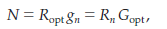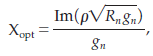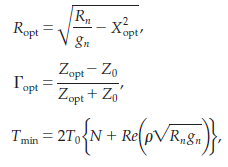(1) Marian W. Pospieszalski in his MTT Microwave Magazine article on "Interpreting Transistor Noise," IEEE Microwave Magazine, vol. 11, no. 6, pp. 61-69, Oct. 2010. doi: 10.1109/MMM.2010.937733

The details of the derivation of Lane’s noise factor equation and the relationship of the Noise Parameters to the circuit elements and noise sources of any linear two-port circuit are somewhat involved, and I refer you to the references in Marian’s paper. The math details are relatively straight-forward but extremely tedious.

A Simple Example

Well, not exactly. I wanted to finish up with a simple example but you would all fall asleep under the crushing tedium of the math – even for a simple FET model with just a dependent current source, a gate capacitor and resistor, and a drain resistor. So, instead, I’m showing you the brilliant work of Joe Bardin, who modeled an IBM SiGe HBT and used the basic methods shown above to predict with great accuracy the noise parameters (and S-parameters) of the SiGe device analytically. Here is the model he used, going so far as to break down the base current and collector current into forward and reverse components. The model includes the noisy resistors RB, RE, and RC, plus the shot noise currents for the base and collector current noise contributions.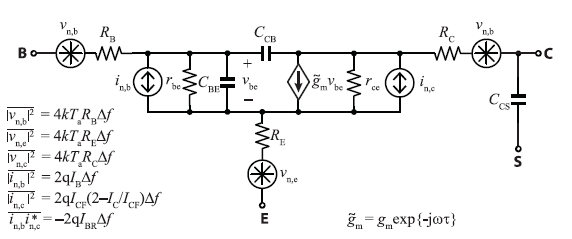And here after a lot of fussy math are the analytically derived noise parameters for this model – check the paper cited below for definitions and approximations.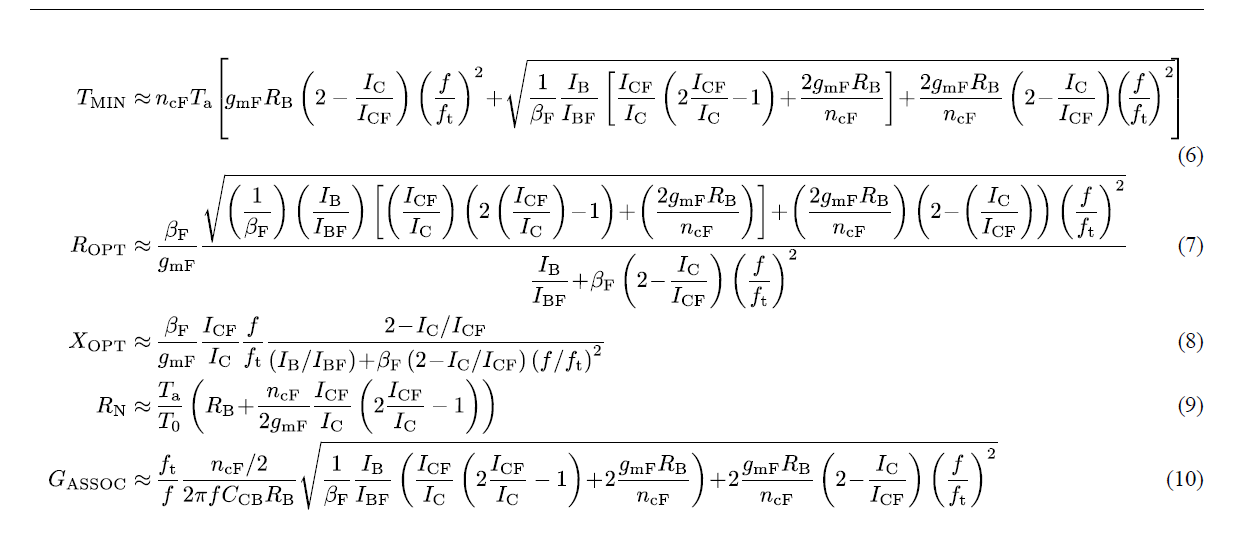And lastly, the proof that all this theory really works on real pieces of hardware. Here is the Noise figure of the transistor plotted versus frequency showing measured and computed performance.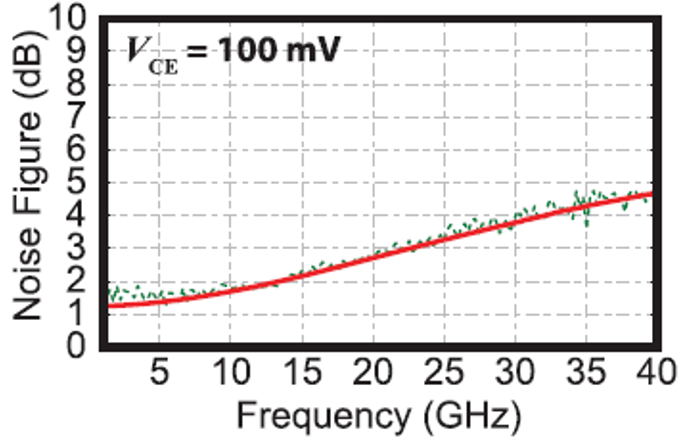S. Montazeri, W. Wong, A. H. Coskun and J. C. Bardin, "Ultra-Low-Power Cryogenic SiGe Low-Noise Amplifiers: Theory and Demonstration," in IEEE Transactions on Microwave Theory and Techniques, vol. 64, no. 1, pp. 178-187, Jan. 2016. doi: 10.1109/TMTT.2015.2497685

Keywords: Johnson noise, Shot noise, Flicker noise, Thermal noise, FET channel noise, Noise Factor, Noise Figure, Lane Optimum Noise Match, Pospieszalski Noise Model, Noise Parameters.

Author : The Other Editor